3.MD.3.-Test1B Solve one- and two-step “how many more”- “how many less” problem
 Name:    3.MD.3.-Test1B Solve one- and two-step “how many more”- “how many less” problem

Multiple Choice
Identify the choice that best completes the statement or answers the question.

1.

According to the graph, how many black cats are there?a. 3 c. 4 b. 6 d. 5

2.

According to the graph, which type of fish are there the least of in the tank?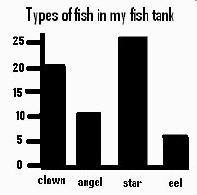a. eel c. angel b. star d. clown

3.

According to the graph which type of fish are there the most of in the tank?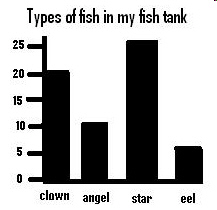a. eel c. star b. angel d. clown

4.

According to the graph, how many star fish are in the tank?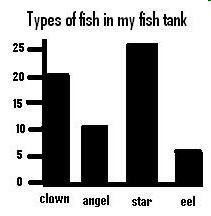a. 5 c. 25 b. 20 d. 10

5.

According to the graph, how many clown fish are in the fish tank?a. 5 c. 25 b. 20 d. 10

6.

According to the graph, how many more white cats are there than tan cats?a. 7 c. 1 b. 2 d. 10

7.

According to the graph, how many cats were counted all together?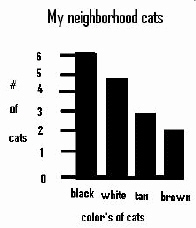a. 12 c. 16 b. 10 d. 19

8.

According to the graph, how many more black cats are there than white cats?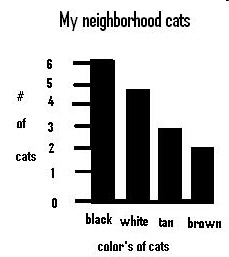a. 2 c. 4 b. 1 d. 6

9.

According to the graph, how many tan cats are there?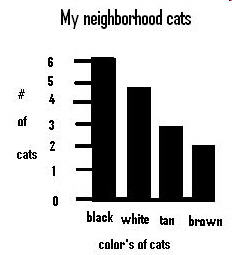a. 6 c. 4 b. 2 d. 3

10.

According to the graph, how many clown and angel fish are there in the tank?a. 30 c. 20 b. 60 d. 10